# Cách xác định sai số của phép đo gián tiếp

How to determine the error of indirect measurement

## Wiki on How to determine the error of an indirect measurement

` Cách xác định sai số của phép đo gián tiếp -`

Question: How to determine the error of indirect measurement

A. The absolute error of a sum or a difference is equal to the sum of the absolute errors of the terms.

B. The absolute error of a sum or a difference is equal to the product of the absolute errors of the terms.

C. The absolute error of a sum or a difference is equal to the quotient of the absolute error of the terms.

D. The absolute error of a sum or a difference is equal to the absolute error of the largest term.

The absolute error of a sum or a difference is equal to the sum of the absolute errors of the terms.

How to determine the error of an indirect measurement:

– The absolute error of a sum or difference is equal to the sum of the absolute errors of the terms.

– The relative error of the product or quotient is equal to the sum of the relative errors of the factors.

– If there are constants in the physical formula for determining an indirect measurand, then that constant must be reduced to a decimal less than 1/10 of the total error contained in the same calculation formula.

– If the formula for determining the indirect measurand is relatively complex and the direct measuring instruments have relatively high accuracy, the error of the instrument can be ignored.

F = x + yz

ΔF = x + y + z

Join Tran Hung Dao High School to learn more about the error of the measurement of physical quantities!

## I. Measurement of physical quantities

The measurement of a physical quantity is the comparison of it with a quantity of the same type that is conventionally used as a unit.

– The direct comparison through the measuring instrument is called direct measurement.

– The determination of a physical quantity through a formula that relates directly to measurands is called indirect measurement.

## II. Measure

The unit of measurement commonly used in the SI system of units.

– The SI system of units is a system of units for measuring physical quantities that has been uniformly regulated and applied in many countries around the world.

The SI system specifies 7 basic units:

+ Length: meters (m)

+ Temperature: Kelvin (K)

+ Time: seconds

+ Amperage: ampere (A)

+ Weight: kilograms (kg)

+ Luminous intensity: candela (Cd)

+ Amount of substance: mole (mol)

## III. Measurement error

1. Error types

a) System error

Is the bias due to the odd part of the device’s accuracy being unreadable (known as device error ΔA’) or the initial zero being skewed.

Instrumentation error ΔA’ is generally considered to be one half or one division on the instrument.

b) Coincidence error

It is a deviation due to limited human perception ability due to the impact of external coincidental factors.

2. Average value

The average value of multiple measurements of a quantity A is calculated: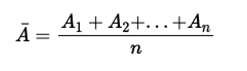This is the closest approximation to the true value of quantity A.

3. How to identify measurement error

– The absolute error for each measurement is the absolute value of the difference between the mean value and the value of each measurement.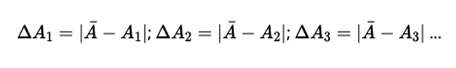– The average absolute error of n measurements is called coincidence error and is calculated: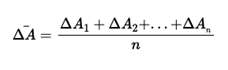– The absolute error of the measurement is the sum of the coincidence error and the means error: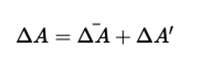– where the tool error ΔA ′ can be taken as one half or one smallest division on the tool.

4. Scale error

The relative error A of a measurement is the ratio of the absolute error to the mean value of the measurand, expressed as a percentage.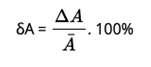5. How to determine the error of indirect measurement

– The absolute error of a sum or difference is equal to the sum of the absolute errors of the terms.

– The relative error of the product or quotient is equal to the sum of the relative errors of the factors.

– If there are constants in the physical formula for determining an indirect measurand, then that constant must be reduced to a decimal less than 1/10 of the total error contained in the same calculation formula.

– If the formula for determining the indirect measurand is relatively complex and the direct measuring instruments have relatively high accuracy, the error of the instrument can be ignored.

Posted by: Tran Hung Dao High School

[rule_{ruleNumber}]

#How to #determine #wrong #number of #measurement #indirect #

[rule_3_plain]

#How to #determine #wrong #number of #measurement #indirect #

Question: How to determine the error of indirect measurement
A. The absolute error of a sum or a difference is equal to the sum of the absolute errors of the terms .
B. The absolute error of a sum or a difference is equal to the product of the absolute errors of the terms .
C. The absolute error of a sum or a difference is equal to the quotient of the absolute errors of the terms .
D. The absolute error of a sum or a difference is equal to the absolute error of the largest term.
The absolute error of a sum or a difference is equal to the sum of the absolute errors of the terms .

How to determine the error of an indirect measurement:
– The absolute error of a sum or difference is equal to the sum of the absolute errors of the terms.
– The relative error of a product or quotient is equal to the sum of the relative errors of the factors.
– If in the physical formula defining indirect measurands there are constants, the constant must be taken to the decimal fraction less than 1/10 of the sum of the errors present in the same calculation formula.
– If the formula for determining the indirect measurand is relatively complex and the direct measuring instruments have relatively high accuracy, the instrument error can be ignored.
F=x+y-z
→ΔF=Δx+Δy+Δz
Join Tran Hung Dao High School to learn more about the error of the measurement of physical quantities!
Quick view of content1 I. Measurement of physical quantities2 II. Unit of measure 3 III. Measurement error
I. Measurement of physical quantities
– The measurement of a physical quantity is the comparison of it with a quantity of the same type that is conventionally used as a unit.
– The direct comparison through the measuring instrument is called direct measurement.
– The determination of a physical quantity through a formula that relates directly measured quantities is called indirect measurement.
II. Unit of measure

The unit of measure commonly used in the SI system of units.
– The SI system of units is a system of units of measurement of physical quantities that has been uniformly regulated and applied in many countries around the world.
The SI system specifies 7 basic units:
+ Length: meters (m)
+ Temperature: kelvin (K)
+ Time: seconds (s)
+ Amperage: ampere (A)
+ Weight: kilograms (kg)
+ Luminous intensity: candela (Cd)
+ Amount of substance: mole (mol)
III. Measurement error
1. Error types
a) Systematic error
Is the deviation due to the odd part of not being able to read the accuracy on the instrument (called the instrument error ΔA’) or the initial 0 point being skewed.
Instrumentation error ΔA’ is usually taken as half or one division on the instrument.
b) Coincidence error
It is a deviation due to the limitation of human sensory ability due to the impact of external coincident factors.
2. Average value
The average value of multiple measurements of a quantity A is calculated:

This is the closest approximation to the true value of quantity A.
3. How to determine measurement error
– The absolute error for each measurement is the absolute value of the difference between the mean value and the value of each measurement.
– The average absolute error of n measurements is called the coincidence error and is calculated:
– The absolute error of the measurement is the sum of the coincident and instrumental errors:
– In which the tool error ΔA′ can be taken as half or one of the smallest divisions on the tool.
4. Ratio error
The relative error A of a measurement is the ratio of the absolute error to the mean value of the measurand, expressed as a percentage.
5. How to determine the error of indirect measurement
– The absolute error of a sum or difference is equal to the sum of the absolute errors of the terms.
– The relative error of a product or quotient is equal to the sum of the relative errors of the factors.
– If in the physical formula defining indirect measurands there are constants, the constant must be taken to the decimal fraction less than 1/10 of the sum of the errors present in the same calculation formula.
– If the formula for determining the indirect measurand is relatively complex and the direct measuring instruments have relatively high accuracy, the instrument error can be ignored.
Posted by: Tran Hung Dao High School

#How to #determine #wrong #number of #measurement #indirect #

[rule_2_plain]

#How to #determine #wrong #number of #measurement #indirect #

[rule_2_plain]

#How to #determine #wrong #number of #measurement #indirect #

[rule_3_plain]

#How to #determine #wrong #number of #measurement #indirect #

Question: How to determine the error of indirect measurement
A. The absolute error of a sum or a difference is equal to the sum of the absolute errors of the terms .
B. The absolute error of a sum or a difference is equal to the product of the absolute errors of the terms .
C. The absolute error of a sum or a difference is equal to the quotient of the absolute errors of the terms .
D. The absolute error of a sum or a difference is equal to the absolute error of the largest term.
The absolute error of a sum or a difference is equal to the sum of the absolute errors of the terms .

How to determine the error of an indirect measurement:
– The absolute error of a sum or difference is equal to the sum of the absolute errors of the terms.
– The relative error of a product or quotient is equal to the sum of the relative errors of the factors.
– If in the physical formula defining indirect measurands there are constants, the constant must be taken to the decimal fraction less than 1/10 of the sum of the errors present in the same calculation formula.
– If the formula for determining the indirect measurand is relatively complex and the direct measuring instruments have relatively high accuracy, the instrument error can be ignored.
F=x+y-z
→ΔF=Δx+Δy+Δz
Join Tran Hung Dao High School to learn more about the error of the measurement of physical quantities!
Quick view of content1 I. Measurement of physical quantities2 II. Unit of measure 3 III. Measurement error
I. Measurement of physical quantities
– The measurement of a physical quantity is the comparison of it with a quantity of the same type that is conventionally used as a unit.
– The direct comparison through the measuring instrument is called direct measurement.
– The determination of a physical quantity through a formula that relates directly measured quantities is called indirect measurement.
II. Unit of measure

Xem thêm bài viết hay:  Dĩa huông là gì? Tại sao dĩa huông lại được giới trẻ dùng nhiều đến vậy?

The unit of measure commonly used in the SI system of units.
– The SI system of units is a system of units of measurement of physical quantities that has been uniformly regulated and applied in many countries around the world.
The SI system specifies 7 basic units:
+ Length: meters (m)
+ Temperature: kelvin (K)
+ Time: seconds (s)
+ Amperage: ampere (A)
+ Weight: kilograms (kg)
+ Luminous intensity: candela (Cd)
+ Amount of substance: mole (mol)
III. Measurement error
1. Error types
a) Systematic error
Is the deviation due to the odd part of not being able to read the accuracy on the instrument (called the instrument error ΔA’) or the initial 0 point being skewed.
Instrumentation error ΔA’ is usually taken as half or one division on the instrument.
b) Coincidence error
It is a deviation due to the limitation of human sensory ability due to the impact of external coincident factors.
2. Average value
The average value of multiple measurements of a quantity A is calculated:

This is the closest approximation to the true value of quantity A.
3. How to determine measurement error
– The absolute error for each measurement is the absolute value of the difference between the mean value and the value of each measurement.
– The average absolute error of n measurements is called the coincidence error and is calculated:
– The absolute error of the measurement is the sum of the coincident and instrumental errors:
– In which the tool error ΔA′ can be taken as half or one of the smallest divisions on the tool.
4. Ratio error
The relative error A of a measurement is the ratio between the absolute error and the mean value of the measurand, expressed as a percentage.
5. How to determine the error of indirect measurement
– The absolute error of a sum or difference is equal to the sum of the absolute errors of the terms.
– The relative error of a product or quotient is equal to the sum of the relative errors of the factors.
– If in the physical formula defining indirect measurands there are constants, the constant must be taken to the decimal fraction less than 1/10 of the sum of the errors present in the same calculation formula.
– If the formula for determining the indirect measurand is relatively complex and the direct measuring instruments have relatively high accuracy, the instrument error can be ignored.
Posted by: Tran Hung Dao High School